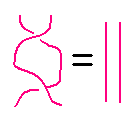occasional meanderings in physics' brave new world

Saturday, April 03, 2010

M Theory Lesson 310

Permutations may be represented by symmetric braids. A category theorist would usually draw a diagram likeindicating that the braid crossing goes neither over nor under, and that one can slide strands across each other. It would be nicer if we could draw a braid likebecause this obviously untangles to the identity braid. However, the two crossings here are different, like in the law $(\sigma) \circ (- \sigma) = 1$, which is to say that $\sigma$ is a bit like the complex $i$, satisfying $i + i^{-1} = 0$. How about elements of $S_3$? The permutation $(231)$ cubes to the identity, as in the diagramwhere the crossings have been chosen so that no strand actually links with any other. Note that each $(231)$ section is a Bilson-Thompson braid. What fun! By assigning $n$-th roots of unity to each strand one obtains a fun operator, for $S_d$. The choice $n = d+1$ (see last time) gives a representation of $S_n$.Kea said...

Recall that the 3d MUB Pauli operators involve cubed roots. We could think of such root labels on three strands as possible half twists in each ribbon strand, and then the Bilson-T charge pieces would be represented by these cubed roots. A typical MUB operator would have a charge set +-0.

April 03, 2010 1:19 PMCarlBrannen said...

I'm starting to think I understand how to get GR from Pauli MUBs. Basically, if you consider one basis you get zitterbewegung and therefore the Dirac equation. This is a 1-dimensional theory; a particle having positive velocity spends more time going right (+c) than left (-c). The time spent going R and L sums up to 1: R+L = 1, while v = R-L.

When you go to three MUBs the situation complicates. Now, instead of balancing between +x and -x, you balance between +x,+y,+z,-x,-y,-z. Thus it is possible for any single dimension to be offset. This fits in perfectly with the Gullstrand-Painleve coordinates for the black hole.

April 03, 2010 2:02 PMKea said...

Oops, I should have mentioned those 6x6 matrices made from putting 2d Pauli ops into a 3x3 circulant ... which is your way of getting the particles ...

Hmm, so you're heading towards a 'Three Time' point of view now? This sounds hopeful ...

April 03, 2010 2:24 PMJason Padgett said...

Hi Marni,

We talked a couple years ago about my fractal drawings. I just wanted to show you some new ones that may help people when they are trying to visualize space time (at the Planck Particle size frame). Here is a link to one of my fractals. It is a time lapse movie of the drawing. I then added color with a computer and a wave equation to make it warp and stretch. I hope you enjoy it. Below is the link.